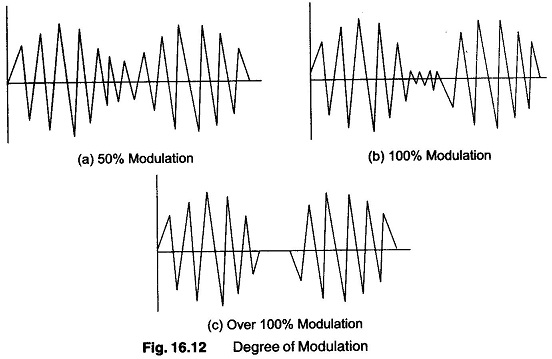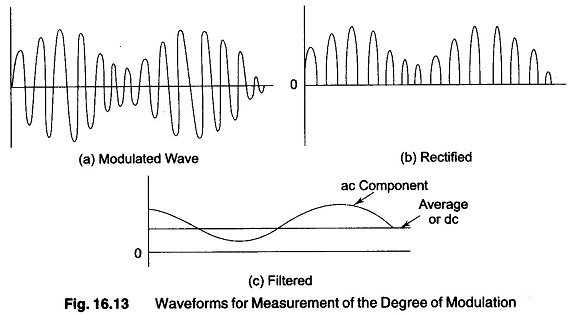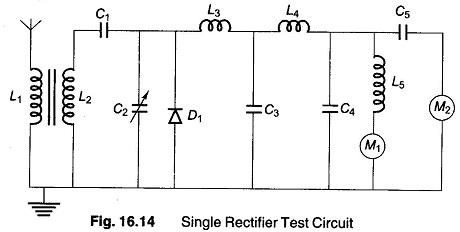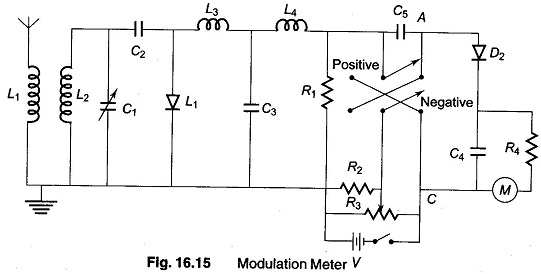## Modulation Frequency Definition:

Modulation Frequency Definition is the process of impressing information on a carrier wave by altering either its amplitude of frequency. In amplitude modulation, the degree is the percentage of amplitude change from the unmodulated value of the carrier.

In Modulation Frequency Definition, the frequency deviation is the change in frequency in one direction from the centre frequency of the carrier. The degree of modulation is important, because it has a direct bearing on output power and band width.

A carrier wave has 100% modulation when the total amplitude change from the crest to the trough is equal to twice the amplitude of the unmodulated carrier. 50% modulation is present when the amplitude of the trough is half the amplitude of the unmodulated carrier. When the amplitude of the trough of a wave reaches zero amplitude and remains there for a finite time period, the wave is over 100% modulated. These three conditions are illustrated in Fig. 16.12.The percentage modulation of a modulated carrier is calculated by dividing the change in amplitude by the amplitude of the unmodulated carrier, multiplied by 100.

One method of measuring the degree of modulation makes use of a wave component separation and comparison test circuit. This circuit rectifies and filters the modulated waves and compares the ac and dc components of the resulting waveform. This process is illustrated in Fig. 16.13.The amplitude of the dc component is proportional to the carrier amplitude. The sine wave amplitude is proportional to the amplitude of the modulated signal.

A basic test circuit arrangement for measuring the degree of modulation is shown in Fig. 16.14.The modulated RF carrier is picked up on the antenna and rectified by the diode. The coils and capacitors filter out the RF components and leave an audio signal riding on the dc component. The meters indicate the values of the ac and dc components. M1 is the dc meter, whose indication is proportional to the amplitude of the unmodulated carrier. M2 is an ac meter whose indication is proportional to the amplitude of the modulating signal.

Since the ac meter reads effective values, 100% modulation causes the reading on M2 to be 0.707 of the reading on M1. This shows the modulating changes in amplitude is equal to twice the amplitude of the unmodulated carrier.

With 50% modulation, the carrier amplitude is equal to the modulating change in the amplitude; in this case the reading on meter M1 is nearly thrice the value of M2.

The circuit of Fig. 16.14 can be modified to provide a continuous reading directly in percentage of modulation, as illustrated in Fig. 16.15.This circuit rectifies the difference in voltage between points A and C. This causes a current through the meter proportional to the percentage of modulation. The meter is calibrated directly in percentage modulation.

### Measuring Frequency Modulation

In FM modulation, the carrier amplitude remains constant and the modulated signal causes the carrier frequency to change.

Using the unmodulated carrier frequency as the reference, the modulated signal frequency varies about the reference frequency. The rate of deviation is the number of total changes per second, and is the same as the frequency of the modulating signal. The degree of deviation is the amount of frequency shift to either side of the reference frequency. The degree of deviation determines the band width of the transmitted signal and the number of sidebands it contains. In FM, all the intelligence is in the sidebands and it is desirable to retain as many sidebands as possible. The Federal Communication Commission allows a maximum deviation of 75 kHz on entertainment broadcast bands. This is narrow band FM transmission. Broad band FM transmission is allowed a much wider frequency deviation, up to several hundred MHz. The maximum allow­able deviation is often referred to as 100% modulation.

Scroll to Top